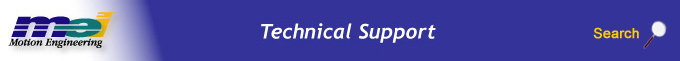.

mpiFilterGainGet

Declaration

 `long mpiFilterGainGet(MPIFilter filter, long gainIndex, MPIFilterGain *gain)` Required Header: stdmpi.h

Description

mpiFilterGainGet gets the gain coefficients of a Filter (filter, for the gain index specified by gainIndex) and writes them into the structure pointed to by gain. Post filters are set using the meiFilterPostfilterGet/Set and meiFilterPostfilterSectionGet/Set methods.

 filter A handle to a Filter object. gainIndex The index number of the filter gain table. Unless you are using gain tables, set this value to 0. If you are setting gain tables in Motion Console, this is the drop down box in Filter Summary->Coeffs that says "Gain Table 0", "Gain Table 1", etc. In standard firmware, the gain table indexes are in the following order: MEIFilterGainIndexNO_MOTION = 0, MEIFilterGainIndexACCEL = 1, MEIFilterGainIndexDECEL = 2, and MEIFilterGainIndexVELOCITY = 3 To see information on gain indexes in non-standard firmware, see MEIFilterGainIndex. *gain Pointer to the gain structure. The gain structure holds the closed loop filter gains. The PID and PIV gains are both set here. The only difference in setting PID vs. PIV gains are the names for the gain indices (see PID example below).

 Return Values MPIMessageOK

Sample Code

 ```/* Sets reasonable tuning parameters for a Trust TA9000 test stand */void setPIDs(MPIFilter filter){ MPIFilterGain gain; long returnValue; returnValue = mpiFilterGainGet(filter, 0, &gain); msgCHECK(returnValue); gain.coeff[MEIFilterGainPIDCoeffGAIN_PROPORTIONAL].f = (float)100; gain.coeff[MEIFilterGainPIDCoeffGAIN_INTEGRAL].f = (float)0.2; gain.coeff[MEIFilterGainPIDCoeffGAIN_DERIVATIVE].f = (float)1000; gain.coeff[MEIFilterGainPIDCoeffFEEDFORWARD_POSITION].f = (float)0; gain.coeff[MEIFilterGainPIDCoeffFEEDFORWARD_VELOCITY].f = (float)45; gain.coeff[MEIFilterGainPIDCoeffFEEDFORWARD_ACCELERATION].f = (float)101000; gain.coeff[MEIFilterGainPIDCoeffFEEDFORWARD_FRICTION].f = (float)450; gain.coeff[MEIFilterGainPIDCoeffINTEGRATIONMAX_MOVING].f = (float)15000; gain.coeff[MEIFilterGainPIDCoeffINTEGRATIONMAX_REST].f = (float)15000; gain.coeff[MEIFilterGainPIDCoeffDRATE].f = (float)0; gain.coeff[MEIFilterGainPIDCoeffOUTPUT_LIMIT].f = (float)32767; gain.coeff[MEIFilterGainPIDCoeffOUTPUT_LIMITHIGH].f = (float)32767; gain.coeff[MEIFilterGainPIDCoeffOUTPUT_LIMITLOW].f = (float)-32767; gain.coeff[MEIFilterGainPIDCoeffOUTPUT_OFFSET].f = (float)0; gain.coeff[MEIFilterGainPIDCoeffNOISE_POSITIONFFT].f = (float)0; gain.coeff[MEIFilterGainPIDCoeffNOISE_FILTERFFT].f = (float)0; gain.coeff[MEIFilterGainPIDCoeffNOISE_VELOCITYFFT].f = (float)0; returnValue = mpiFilterGainSet(filter, 0, &gain); msgCHECK(returnValue);}``` ------------------ Another way to change filter coefficients is to use mpiFilterConfigGet /Set. ``` returnValue = mpiFilterConfigGet(filter, &config, NULL); msgCHECK(returnValue); /* Look in MEIFilterGainPIDCoeff to get the indexes. Not all of the above coefficients are shown in this short example. */ config.gain.coeff[MEIFilterGainPIDCoeffGAIN_PROPORTIONAL].f = (float)100; config.gain.coeff[MEIFilterGainPIDCoeffGAIN_INTEGRAL].f = (float)0.2; config.gain.coeff[MEIFilterGainPIDCoeffGAIN_DERIVATIVE].f = (float)1000; returnValue = mpiFilterConfigSet(filter, &config, NULL); msgCHECK(returnValue); ```